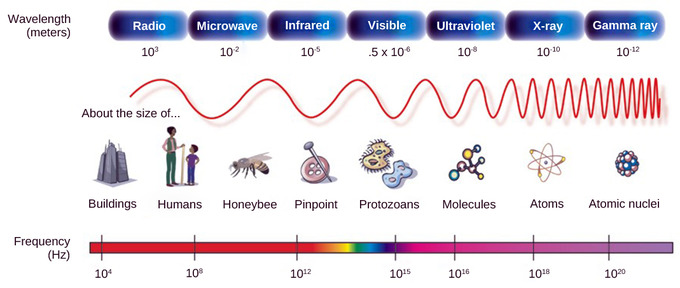# 36.5A: Light

•• Boundless
• Boundless
$$\newcommand{\vecs}{\overset { \rightharpoonup} {\mathbf{#1}} }$$ $$\newcommand{\vecd}{\overset{-\!-\!\rightharpoonup}{\vphantom{a}\smash {#1}}}$$$$\newcommand{\id}{\mathrm{id}}$$ $$\newcommand{\Span}{\mathrm{span}}$$ $$\newcommand{\kernel}{\mathrm{null}\,}$$ $$\newcommand{\range}{\mathrm{range}\,}$$ $$\newcommand{\RealPart}{\mathrm{Re}}$$ $$\newcommand{\ImaginaryPart}{\mathrm{Im}}$$ $$\newcommand{\Argument}{\mathrm{Arg}}$$ $$\newcommand{\norm}{\| #1 \|}$$ $$\newcommand{\inner}{\langle #1, #2 \rangle}$$ $$\newcommand{\Span}{\mathrm{span}}$$ $$\newcommand{\id}{\mathrm{id}}$$ $$\newcommand{\Span}{\mathrm{span}}$$ $$\newcommand{\kernel}{\mathrm{null}\,}$$ $$\newcommand{\range}{\mathrm{range}\,}$$ $$\newcommand{\RealPart}{\mathrm{Re}}$$ $$\newcommand{\ImaginaryPart}{\mathrm{Im}}$$ $$\newcommand{\Argument}{\mathrm{Arg}}$$ $$\newcommand{\norm}{\| #1 \|}$$ $$\newcommand{\inner}{\langle #1, #2 \rangle}$$ $$\newcommand{\Span}{\mathrm{span}}$$

Light is composed of photons that make up electromagnetic waves, which are characterized by wavelength, frequency, and amplitude.

Learning Objectives

• Describe the characteristics of light

## Key Points

• Light is composed of electromagnetic waves that can travel without a medium, unlike sound.
• The behavior of light can be seen in the behavior of waves and photons, the basic unit of light.
• A wavelength (which varies inversely with frequency ) manifests itself as color, while wave amplitude is perceived as luminous intensity or brightness; it is measured by the standard unit of a candela.
• Humans can see light that ranges between 380 nm and 740 nm, but cannot see light that is below the frequency of visible red light or above the frequency of visible violet light.
• Light at the red end of the visible spectrum has long wavelengths (and is lower frequency), while light at the violet end has short wavelengths (and is higher frequency).
• Light waves enter the eye as long (red), medium (green), and short (blue) waves; the color of an object is the color the object reflects.

## Key Terms

• photon: the quantum of light and other electromagnetic energy, regarded as a discrete particle having zero rest mass, no electric charge, and an indefinitely long lifetime
• nanometer: one billionth of a meter; used to express wavelength of light
• electromagnetic spectrum: the entire range of wavelengths of all known radiations consisting of oscillating electric and magnetic fields, including gamma rays, visible light, infrared, radio waves, and X-rays
• wavelength: the length of a single cycle of a wave, as measured by the distance between one peak or trough of a wave and the next; it corresponds to the velocity of the wave divided by its frequency

## Light

As with auditory stimuli, light travels in waves. While the compression waves that compose sound must travel in a medium (consisting of a gas, a liquid, or a solid), light is composed of electromagnetic waves and needs no medium. Light can, in fact, travel in a vacuum. The behavior of light can be described in terms of the behavior of waves and the behavior of the fundamental unit of light, the photon: a packet of electromagnetic radiation. A glance at the electromagnetic spectrum shows that visible light for humans is just a small slice of the entire spectrum, which includes radiation that we cannot see as light because it is below the frequency of visible red light and above the frequency of visible violet light.Figure $$\PageIndex{1}$$: Electromagnetic spectrum: A glance at the electromagnetic spectrum shows that visible light for humans is just a small slice of the entire spectrum.

Certain variables are important when discussing perception of light. A wavelength (which varies inversely with frequency) manifests itself as color. Light at the red end of the visible spectrum has longer wavelengths (and is lower frequency), while light at the violet end has shorter wavelengths (and is higher frequency). The wavelength of light is expressed in nanometers (nm); one nanometer is one billionth of a meter. Humans perceive light that ranges between approximately 380 nm and 740 nm. However, some other animals can detect wavelengths outside of the human range. For example, bees see near-ultraviolet light in order to locate nectar guides on flowers. Some non-avian reptiles sense infrared light (such as heat that prey gives off).

Wave amplitude is perceived as luminous intensity or brightness. The standard unit of intensity of light is the candela, which is approximately the luminous intensity of one common candle.

Light waves travel 299,792 km per second in a vacuum and somewhat slower in various media such as air and water. Those waves arrive at the eye as long (red), medium (green), and short (blue) waves. The term “white light” is light that is perceived as white by the human eye. This effect is produced by light that stimulates the color receptors in the human eye equally. The apparent color of an object is actually the color (or colors) the object reflects. Thus a red object reflects the red wavelengths in mixed (white) light and absorbs all other wavelengths of light.

36.5A: Light is shared under a not declared license and was authored, remixed, and/or curated by Boundless.